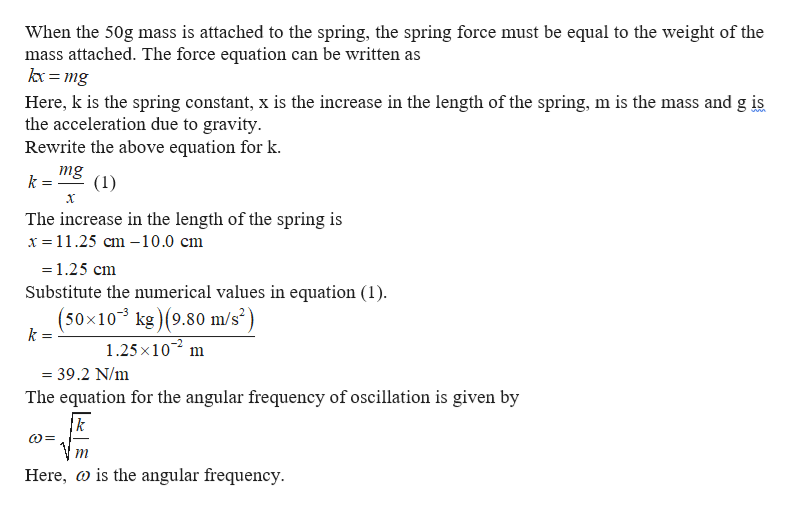# A very light spring is 10.0 ?? long with no mass attached to it. When 50.0 grams are added to it, its length increases to 11.25 ??. Another 100.0 − ? mass is added to the, and it is set into oscillation. How much time is required for 100 complete oscillations

Question
30 views

A very light spring is 10.0 ?? long with no mass attached to it. When 50.0 grams are added to it, its length increases to 11.25 ??. Another 100.0 − ? mass is added to the, and it is set into oscillation. How much time is required for 100 complete oscillations

check_circle

Step 1help_outlineImage TranscriptioncloseWhen the 50g mass is attached to the spring, the spring force must be equal to the weight of the mass attached. The force equation can be written as kx mg Here, k is the spring constant, x is the increase in the length of the spring, m is the mass and g is the acceleration due to gravity Rewrite the above equation for k mg k = (1) The increase in the length of the spring is x 11.25 cm -10.0 cm =1.25 cm Substitute the numerical values in equation (1). (50x103 kg) (9.80 m/s) k 1.25x 10 m =39.2 N/m The equation for the angular frequency of oscillation is given by k is the angular frequency Here, fullscreen

### Want to see the full answer?

See Solution

#### Want to see this answer and more?

Solutions are written by subject experts who are available 24/7. Questions are typically answered within 1 hour.*

See Solution
*Response times may vary by subject and question.
Tagged in